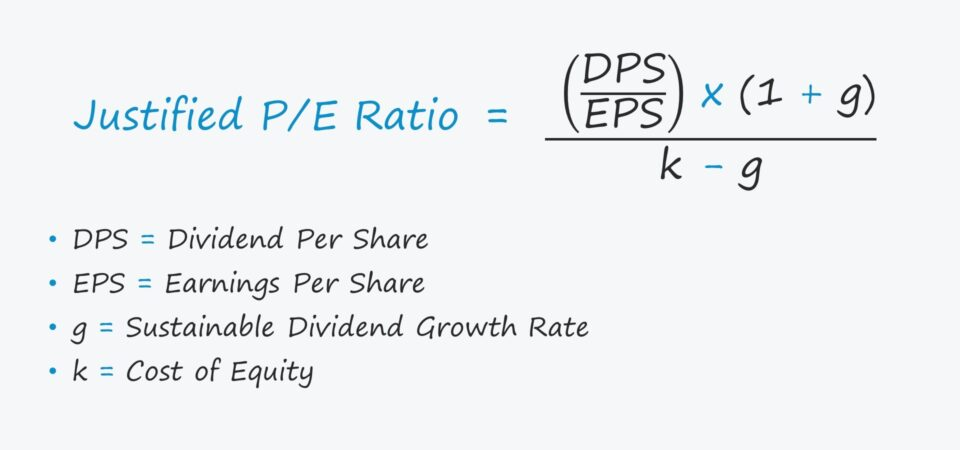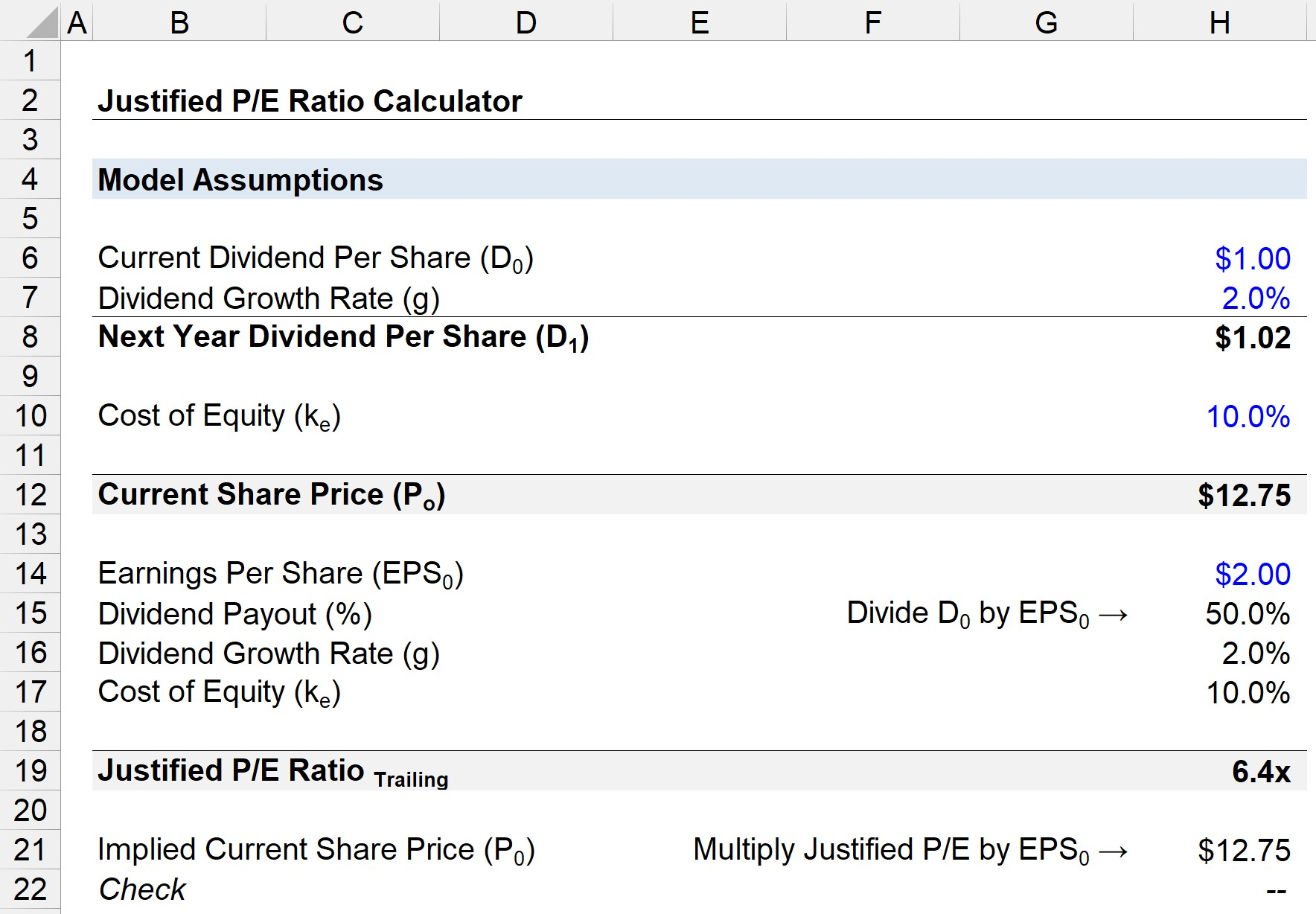Welcome to Wall Street Prep! Use code at checkout for 15% off.# Justified P/E Ratio

Understand the Justified P/E Ratio## How to Calculate Justified P/E Ratio?

The justified P/E ratio can be thought of as an adjusted variation of the traditional price-to-earnings ratio that aligns with the Gordon Growth Model (GGM).

The Gordon Growth Model (GGM) states that a company’s share price is a function of its next dividend payment divided by its cost of equity less the long-term sustainable dividend growth rate.

Current Share Price (Po) = [Do * (1 + g)] / (k – g)

Where:

Moreover, if we divide both sides by the EPS – the current share price and the dividend per share (DPS) – we are left with the justified P/E ratio.

## Justified P/E Ratio Formula

The formula to calculate the justified P/E ratio is as follows.

Justified P/E Ratio = [(DPS / EPS) * (1 + g)] / (k – g)

Note how the “(DPS / EPS)” component is the dividend payout ratio %.

Since the payout ratio is expressed in the form of a percentage, the GGM formula is effectively converted into the justified P/E ratio.

• Trailing: If the EPS used is the current period historical EPS, the justified P/E is on a “trailing” basis.
• Forward: If the EPS used is the forecasted EPS for a future period, the justified P/E is on a “forward” basis.

## Core Value Drivers of the Justified P/E Ratio

The fundamental drivers that impact the justified P/E are the following:

1) Inverse Relationship with Cost of Equity

• Higher Cost of Equity → Lower P/E
• Lower Cost of Equity → Higher P/E

2) Direct Relationship with Dividend Growth Rate

• Higher Dividend Growth Rate → Higher P/E
• Lower Dividend Growth Rate → Lower P/E

3) Direct Relationship with Dividend Payout Ratio (%)

• Higher Payout Ratio % → Higher P/E
• Lower Payout Ratio % → Lower P/E

Therefore, the justified P/E ratio indicates that a company’s share price should rise from a lower cost of equity, higher dividend growth rate, and higher payout ratio.

## Justified P/E Ratio Calculator – Excel Template

We’ll now move to a modeling exercise, which you can access by filling out the form below.Submitting...

## 1. Current Share Price Calculation Example

Suppose a company paid a dividend per share (DPS) of \$1.00 in the most recent reporting period.

• Dividend Per Share (Do) = \$1.00
• Sustainable Dividend Growth Rate = 2%

As for the rest of our model assumptions, the company’s cost of equity is 10% and the sustainable dividend growth rate is 2.0%

• Dividend Growth Rate (g) = 2%
• Cost of Equity (ke) = 10%

If we grow the current dividend by the growth rate assumption, the next year’s dividend is \$1.02.

• Next Year Dividend Per Share (D1) = \$1.00 * (1 + 2%) = \$1.02

Using those assumptions, the justified share price comes out as \$12.75.

• Current Share Price (Po) = \$1.02 /(10% – 2%) = \$12.75

## 2. Justified P/E Ratio Calculation Example

In the next part, we will calculate the justified P/E ratio.

However, we are missing one assumption, the reported earnings per share (EPS) of our company in the past year – which we’ll assume was \$2.00.

• Earnings Per Share (EPS) = \$2.00

But if we were to divide both sides by EPS, we can calculate the justified P/E ratio.

• Justified P/E Ratio = [(\$1.00 / \$2.00) * (1 + 2%)] / (10% – 2%) = 6.4x

In closing, we can cross-check the implied share price from the justified P/E and the current share price to ensure our calculation is correct.

After multiplying the justified P/E of 6.4x by the historical EPS of \$2.00, we calculate the implied current share price as \$12.75, which matches the Po from earlier.

• Implied Current Share Price (Po) = 6.4x * \$2.00 = \$12.75Step-by-Step Online Course

### Everything You Need To Master Financial Modeling

Enroll in The Premium Package: Learn Financial Statement Modeling, DCF, M&A, LBO and Comps. The same training program used at top investment banks.